Next: 6.2 Geometrical Representation Up: 6. The Complex Numbers Previous: 6. The Complex Numbers   Index

# 6.1 Absolute Value and Complex Conjugate

6.1   Definition (Complex Numbers,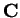.) We denote the complexification of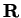by, and we callthe complex numbers.

6.2   Definition (Absolute value.) In exercise 4.23A we showed that (for any field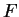in which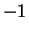is not a square), if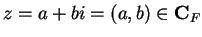, then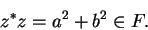If we are working in, then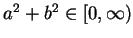and hencehas a unique square root in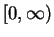, which we denote by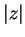and call the absolute value of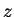.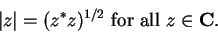We note that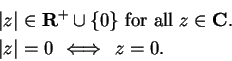Also note that for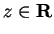, this definition agrees with our old definition of absolute value in.

6.3   Definition (Real and imaginary parts.) Let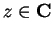and write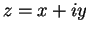where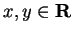. We call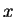the real part of, and we call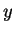the imaginary part of(note that the imaginary part ofis real), and we write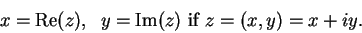6.4   Theorem. Let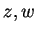be complex numbers. Then
a).
b)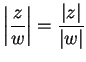if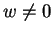.
c)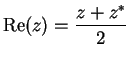.
d)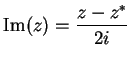.
e)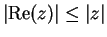.
f)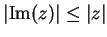.
g)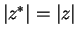.
h).
i)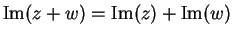.

Proof: By using properties of the complex conjugate proved in exercise 4.23A, we haveHence by uniqueness of square roots,. The proofs of b), c), d), e), f), g), h) and i) are left to you.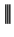6.5   Exercise. Prove parts b), c), d), e), f), g), h) and i) of Theorem 6.4. A

6.6   Theorem (Triangle inequality.) Let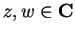. Then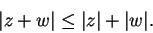Proof: For all,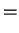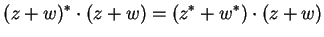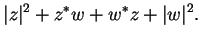(6.7)

Now since, we have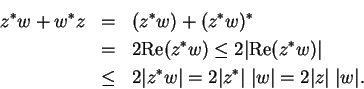Hence, from (6.7),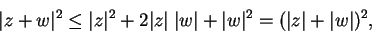and it follows that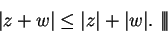Next: 6.2 Geometrical Representation Up: 6. The Complex Numbers Previous: 6. The Complex Numbers   Index